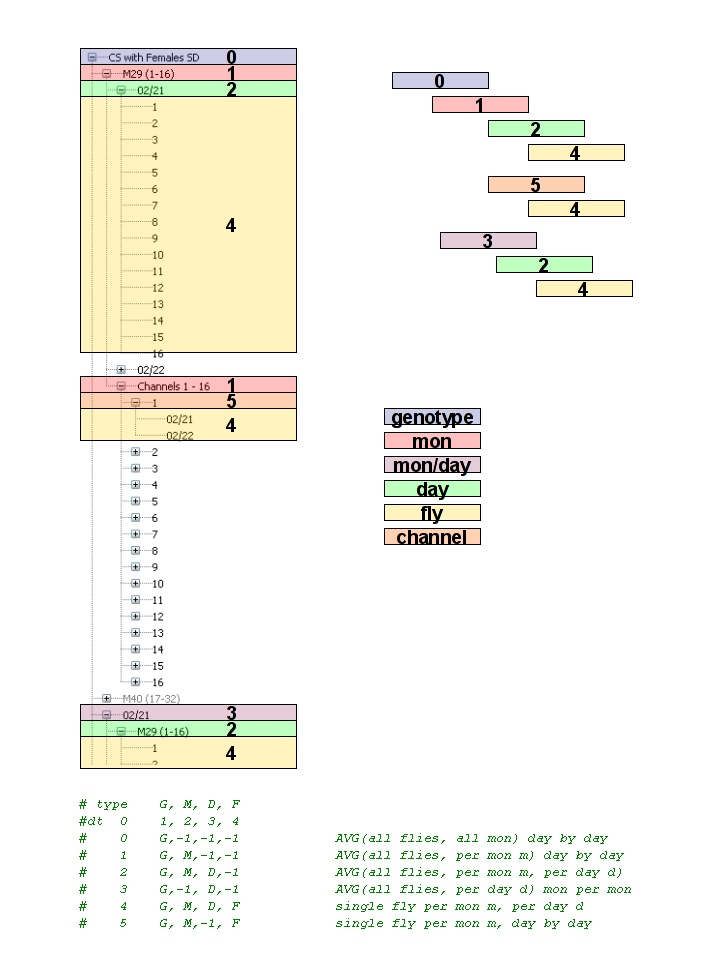A software for sleep analysis in Drosophila

# Portfolio

### The tree coordinates system

Every time the user clicks on the navigation tree, the program knows what data we are supposed to fetch. A sequence of 5 coordinates is then passed to the variable called

GUI[‘dtList’]

and the five coordinates are five integers

t, g, m, d, f

that you will use to retrieve data from the list of DAMslices.

• t = coord_class (optional)
• g = genotype
• m = monitor (not monitor name but monitor number, from 0 to n_m)
• d = day (day number, from 0 to n_d)
• f = fly number (from 0 to n_f)

Normally data are retrieved in the following way:

allSelections = GUI[‘dtList’] #list of lists – all the coordinates of all the selected tree items
t, k, m, d, f = allSelections #we take only the first selection here
cSEL = cDAM[k] #cSEL is the slice we will work with
print cSEL.getGenotype()

The following map shows how the coordinates are passed.The tree at work

So if the user will click on the label that says M29 (namely, the user selects the data for the entire monitor), the values of the coordinates will be:

T = 1
G = 0
M = 0
D = -1
F = -1

-1 indicates we take all available values.

If the user will click on channel 1 of day 2/22 of M29 the values will be:

T = 5
G = 0
M = 0
D = 1
F = 0